# Quadratic equation facts for kids

Kids Encyclopedia Facts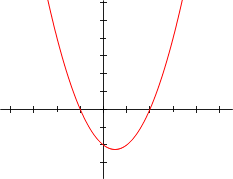A quadratic equation graphed in the coordinate plane.

A quadratic equation is an equation in the form of ax2 + bx + c, where a is not equal to 0. It makes a parabola (a "U" shape) when graphed on a coordinate plane.

The quadratic formula is a formula used to find the points where the graphed equation crosses the x-axis, or the horizontal axis. These points are called the "zeroes" of a function. The formula is: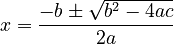$x = \frac{-b \pm \sqrt {b^2-4ac}}{2a}$

Where the letters are the corresponding numbers of the original equation, ax2 + bx + c = 0. Also, a cannot be 0 for the formula to work properly.

The factored form of this equation is y = a(xs)(xt), where s and t are the zeros, a is a constant, and y and the two xs are ordered pairs which satisfy the equation.

### Proof

The quadratic formula is proved by completing the square,

Divide the quadratic equation by a :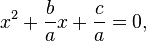$x^2 + \frac{b}{a} x + \frac{c}{a}=0,\,\!$

Move c/a: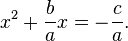$x^2 + \frac{b}{a} x= -\frac{c}{a}.\,\!$

Use the method of completing the square

To "complete the square" is to find some "k" so that:$x^2 + \frac{b}{a} x +k = x^2+2xy+y^2,\,\!$
for some y.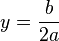$y = \frac{b}{2a}\,\!$
and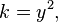$k = y^2,\,\!$
so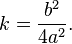$k = \frac{b^2}{4a^2}.\,\!$

Add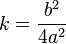$k = \frac{b^2}{4a^2}\,\!$ to both sides of the equation: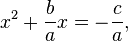$x^2 + \frac{b}{a} x= -\frac{c}{a},\,\!$

Which gives: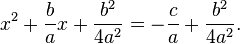$x^2+\frac{b}{a}x+\frac{b^2}{4a^2}=-\frac{c}{a}+\frac{b^2}{4a^2}.\,\!$

The left side is now a perfect square; it is the square of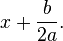$x + \frac{b}{2a}.\,\!$

The right side can be a single fraction, with a common denominator 4a2.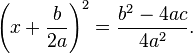$\left(x+\frac{b}{2a}\right)^2=\frac{b^2-4ac}{4a^2}.$

Find the square root of both sides.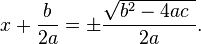$x+\frac{b}{2a}=\pm\frac{\sqrt{b^2-4ac\ }}{2a}.$

Move b/2a: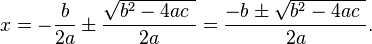$x=-\frac{b}{2a}\pm\frac{\sqrt{b^2-4ac\ }}{2a}=\frac{-b\pm\sqrt{b^2-4ac\ }}{2a}.$Quadratic equation Facts for Kids. Kiddle Encyclopedia.